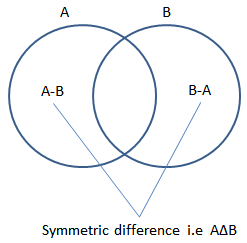# Symmetric Difference Calculator (Calculate A Delta B)

The values of set should be entered with comma seperated example : 1,4,8,9,12

 Enter the elements of the set(A) : Enter the elements of the set(B) :

Symmetric Difference A Δ B :The symmetric difference for two sets A and B can be define as the set of elements which are in either of the sets and not in their intersection. Symmetric difference gives all elements in set A that are not in set B and all elements in set B that are not in set A. It is the union of complement of A with respect to B and B with respect to A. the symmetric difference is commutative and associative. for Example :

Set A = { 2, 8, 9, 9, 13, 17} and

Set B = { 4, 9, 22, 25}

So A Δ B = { 2, 8, 13, 17, 4, 22, 25}